## Indexed and associative Arrays in PHP

Variables are not doubt very efficient in storing temporary data. But each variable can only store one value at a time. So if you are handling a large amount of data Arrays are the best option you have to store and manipulate it. We will be discussing Simple arrays (or indexed arrays) and the Associative arrays in this tutorial. Also the “for each” loop will be discussed since we skipped it last time.

##### Simple or Indexed Arrays- Indexed and associative Arrays in PHP

In PHP, we can simply create a new array using this function “array();”. You need to add all the values inside this function separated by a comma and finally store the whole function to a variable e.g. \$first_Array=array(11,22,44,88,100);

In this example given above, we created a new array with values of 11, 22, 44, 88 and 100. And gave this array a name of \$first_Array. Now why this array called an indexed array is because of the reason that we access all the data inside it using indexes. Index of every array starts from 0. Given is the syntax of accessing an element in an array.

\$arrayName[Index];

Let’s say we want to print the first value of \$firstArray. We will have to write “echo \$first_Array”. This will print the first value i.e. 11. Accessing other elements will be like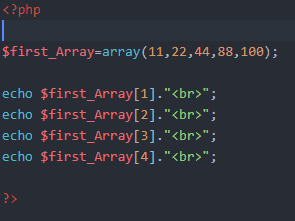Output of this will be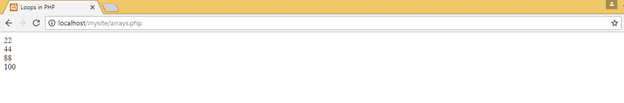One useful function that you must need to remember is count(); It tells the whole length of an array. So in order to make it loop through the whole array this function is really handy. For exampleNow this loop will output all of the entries in an array regardless of the number of entries available in it.

##### Associative Arrays- Indexed and associative Arrays in PHP

These are some special kind of arrays that are used to store a title along with a value. We can access any value by mentioning the title of that entry instead of an index. Let’s look at their syntax

\$assoc_Array= array(“title1”=>”value”, “title2”=>”value”,…). Here is an exampleSo the value of these titles will be printed as

#####For Each Loop- Indexed and associative Arrays in PHP

Last time we skipped this topic as it is purely related to arrays and the best logical way was to discuss it with the arrays. It is really handy in looping through an array of any kind. Here is all you need to know

It is similar to for loop with a minor difference. Syntax to loop through an indexed array is simple

foreach(\$arrayName as \$temp ){

code;

}

Here, temp will hold each value from the array one by one and run the code inside it. We can use the value of temp on each iteration for our purpose. Let’s print the value of an array using this loop.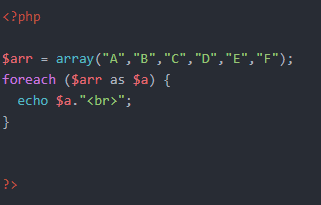In this example the variable \$a will access the values of \$arr and inside the code we will print them. Here is the output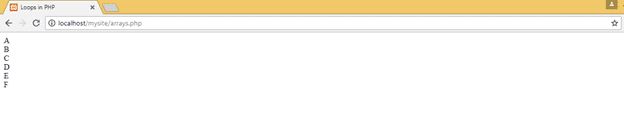There is also another version of this loop to access the values of an associative array. In this loop we mention

Foreach(\$arr as \$title => \$value)

Since there are titles for each value in associative array, hence we make a variable to hold the value of it as well on each iteration. A real example is

#####Indexed and associative Arrays in PHP

And the output of this is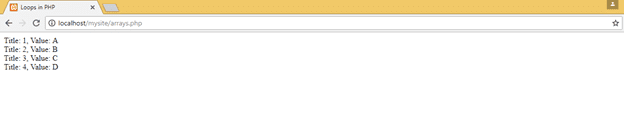We will end this tutorial here. Keep sending us your feedback on these tutorials. For next time we will discuss Functions in PHP. Stay connected!